HOME COURSES PREVIEW REVIEW ABOUT CONTACTTOLL-FREE INFO & ORDERING: M-F: 9am-5pm (PST): (877) RAPID-10

 Quick Search: Keywords:

 Rapid Learning Member Area:Note: If you are a legacy user of chemistry24 members, please request a new login access to the premium server with your full name and old login email via vip@rapidlearningcenter.com

 Rapid Courses Catalog : Physics in 24 Hours Chemistry in 24 Hours Biology in 24 Hours Mathematics in 24 Hours

Weekly Physics Tips:
Want to become a top gun in your class? How about study less yet score high? Sign up this Physics Survival Weekly to learn how. Designed specifically for students who are taking physics, this free newsletter will show you how to survive and excel in class! Weekly topics include:
• How to Study Physics Effectively
• How to Read Physics Textbooks Easily
• How to Solve Physics Problems Systematically
• How to Score High on Physics Exams Strategically
• How to Master Physics Rapidly
Each week, you will receive study tips on the topics above and visual tutorial or study template to enhance your physics learning. Enter your name and email below to subscribe free:

 Physics Study Lounge These study sheets are for quick review on the subjects. Refer to our rapid courses for comprehensive review.     - Basic Skills in Physics     - Basic Math for Physics     - How to Solve Physics Problems     - Newton's Laws Study Guide     - ElectroMagnetism Quick Review     - Atomic Physics At-A-Glance     - Core Concepts in Nuclear Physics     - Special Relativity Overview

 Other Related Sites
 Note: For course links to launch, disable popup blockers or hold the ctrl key while clicking the link.Home » College Calculus

Integration and the Applications

 Topic Review on "Title": The Mean Value Theorem for Integrals: Let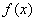be a differentiable and continuous function on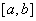. Then the average value is given as:. Basic Integration Rules:Upper and Lower Riemann Sums: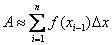(Lower Riemann Sum) where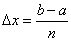for [a,b] and where n is the number of subintervals.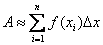(Upper Riemann Sums) wherefor [a,b] and where n is the number of subintervals.

Rapid Study Kit for "Title":
 Flash Movie Flash Game Flash Card Core Concept Tutorial Problem Solving Drill Review Cheat Sheet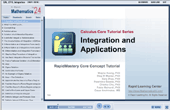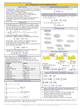"Title" Tutorial Summary : This tutorial introduces basic concepts of integration and builds the foundation for the introduction of the concept of finding the area of a region using Riemann sums and consequently integration. The First and Second Fundamental Theorem of Calculus are presented with the aid of examples. All theorems are discussed with definitions, proof and examples. The Mean Value Theorem as it corresponds to intervals is mentioned in this tutorial with examples. The concept of using a change of variables to evaluate an indefinite or definite integral is given here with an innovative strategy. Determining how to integrate an even or odd function is given with a series of scenarios.

 Tutorial Features: Specific Tutorial Features: Detailed examples to show important concepts such as the Mean Value Theorem for Integrals.. Step by step examples are shown to handle the concept of integrating a definite or an indefinite integral. A classification of what functions are odd or even is given in the form of a table, Series Features: Concept map showing inter-connections of new concepts in this tutorial and those previously introduced. Definition slides introduce terms as they are needed. Visual representation of concepts Animated examples—worked out step by step A concise summary is given at the conclusion of the tutorial.

 "Title" Topic List: `General solution of a differential equation Indefinite integral notation for antiderivatives Basic integration rules to find antidenivativesSigma notation to write an evaluate a sum Area of a plane region Area of a plane region using limitsThe definition of a Riemann SumEvaluating a definite integral using limitsEvaluating a definite integral using properties of definite integralsThe Fundamental Theorem of Calculus and the definite integral The Mean Value of IntegralsThe average value of a function over a closed intervalThe Second Fundamental Theorem of CalculusUsing pattern recognition to evaluate an indefinite integralUse a change of variables to evaluate an indefinite integralUsing the General Power Rule for integration to evaluate an indefinite integralUse a change of variables to evaluate a definite integralEvaluate a definite integral involving an even or odd function`

See all 24 lessons in college calculus, including concept tutorials, problem drills and cheat sheets:
Teach Yourself College Calculus Visually in 24 Hours